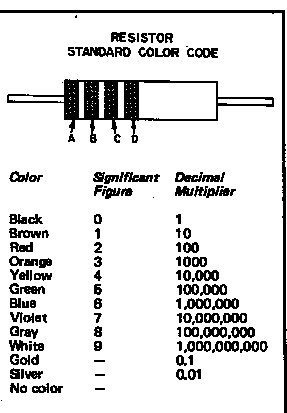Resistors & Capacitors

Recognising resistors and capacitors.Resistor Colour Coding
In most common resistors:
A = 1st figure of the value
B = 2nd figures of the value
C = Number of noughts to add (to make value in Ohms)
D = Tolerance
Gold: 5%, Silver: 10%, none: 20%

Yellow/Violet/Orange = 4700
47000 = 47K

(K is 000, M is 000,000)
Brown/Black/Orange/Gold
Is 10K (5%) etc
.

The European designation is becoming common these days in which the multiplier (K or M) replaces the decimal point. i.e.

 4700 ohms=4.7K ohms - written as 4K7 1M ohm as 1M0 51 ohms as 51R etc.

Capacitor Markings and Values (µ and n etc.)

µ = microfarads, n = nanofarads, p = picafarads. The relationship is easy - the common ones are:

 0.001uF = 1n9(=1000pF) 0.01uF = 10n 0.1uF = 100n

There are a variety of (sometimes obscure) capacitor colour codings but the commonest markings on modern capacitors are:

A three number system where the third number is the number of noughts after the first two - which are the base value.

 101 = 100pF 102 = 0.001uF (1n) 103 = 0.01uF (10n) 104 = 0.1uF (100n)

- are the common ones but watch out for confusion:

 203 = 0.02uF 220 = 22pF 221 = 220pF 4R7 = 4.7pF - etc.

 J = 5% K = 10% M = 20% are the common ones,

There may be a letter to indicate tolerance:

So 474J = 470000 = 0.47uF = 470n